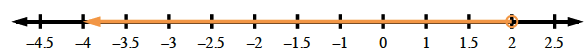### Home > MC2 > Chapter 5 > Lesson 5.2.3 > Problem5-68

5-68.

Graph these inequalities on a number line.

1. $x > 3$

1. $x ≤ 5$

1. $x ≥ –4$

What values could be substituted for $x$ to make the given inequality true?
How can you show all of these values on a number line?
Remember, inequalities with the expression $≥$ or $≤$ have a filled in dot because they include the given point in the expression.

$x < 2$
Values for $x$ that will make the inequality a true statement are: $1.9$, $1.5$, $0$, $−3$, $−10.2$
See graph below.